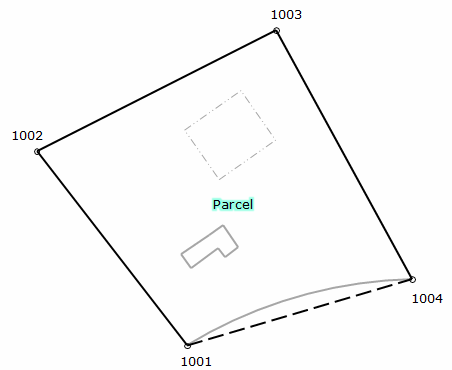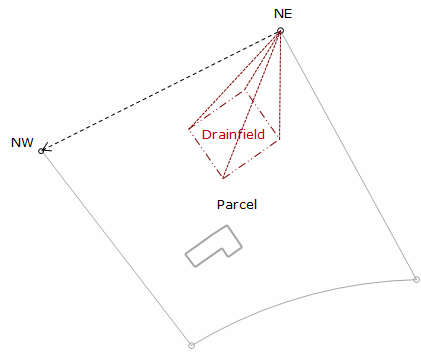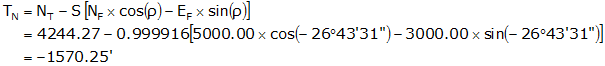## 4. Example

A crew surveyed the exterior of the parcel shown. They assumed the coordinates of the southernmost corner (1001) and used the record bearing for the southwesterly line (1001-1002).Point North East 1001 3684.22 2208.05 1002 4030.04 1940.56 1003 4244.27 2366.04 1004 3802.06 2607.77
Figure H-20
Parcel Survey

Later, a second survey crew was sent out to locate the existing drainfield. They did not have the first crew's coordinates so they occupied the northernmost corner (NE), for which they assumed coordinates, and backsighted the westernmost corner (NW), for which they assumed a direction. They then shot the drainfield corners. As a check, they also shot the distance from NE to NW.Point North East NE 5000.00 3000.00 501 4914.03 2776.03 502 4814.98 2786.38 503 4829.01 2911.57 504 4934.06 2895.69
Figure H-21
Drainfield Survey

When the coordinates were brought together and plotted the results were as shwon in Figure H-22:Figure H-22 Parcel and Drainfield Combined

Using the boundary survey as the reference system, translate the drainfield data.

Determine transformation parameters

Step (1) Rotation angle

Line NE-NW coincides with line 1003-1002; line NE-NW must be rotated into line 1003-1002.Convert the bearings to azimuths and compute the rotation angle.

 Line NE-NWLine 1000-1002From Equation H-1:Step (2) Scale

Length of line NE-NW is 476.41', line 1003-1002 is 476.37'.

From Equation H-2:This represents a distortion of 1/(1-0.999916) = 1/11,900. If this is within tolerance, then the scale factor can be ignored. We will apply it in this example.

Step (3) Translations

Use the coordinates of points 1003 and NE in Equation H-3:Step (4) Math check

For a parameters math check, convert NW to see if it matches the coordinates of 1002.

In the From system, NW's coordinates are:Transform and check:Step (5) Transform points 501-504

Use Equation H-14 with the parameters computed in Steps (1)-(3)

Point 501Point 502Point 503Point 504